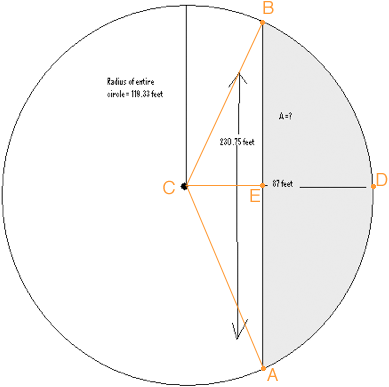SEARCH HOMEMath Central Quandaries & QueriesHi, I need help finding the area of a part of a circle that involves a chord. I need this answer/help very soon if at all possible. I have attached a drawing of what I am trying to solve. Thank you for your help! Sarah!Hi Sarah,You want to find the area of the region you shaded gray in your diagram. I will show you how to find half the area and then you can double it. I am going to find the area of EDB as the area of the sector BCD minus the area of the triangle BCE.

The length of BE is 230.75/2 = 115.375 ft and the length of CE is 119.32 - 87 = 32.32 ft. Hence you can find the area of the triangle BCE using the fact that the area of a triangle is half the base times the height.

All that remains is to find the area of the sector BCD. To determine this area I need the measure of the angle BCD. Tan(∠BCD) = |BE|/|CE| = 115.375/ 32.32 = 3.5698 and hence

∠BCD = tan-1(3.5698) = 74.35o.

The sector BCD is a fraction of the entire circle (π r2) and the angle BCD is a fraction of 360o. By the symmetry these fractions are the same, that is

area(sector BCD)/ (π 119.322 ) = 74.35o/360o

Solve for the area of the sector BCD.

PennyMath Central is supported by the University of Regina and The Pacific Institute for the Mathematical Sciences.